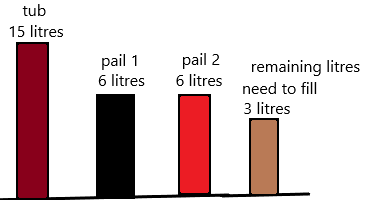Practice the problems of Math in Focus Grade 2 Workbook Answer Key Chapter 9 Key Volume to score better marks in the exam.

Math Journal

Read how Pete solved a real-world problem, Answer, the questions that follow.

Problem
Jug A has 5 liters of lemonade.
Jug B has 3 liters of lemonade.
Simon pours more lemonade into Jug B.
Now, Jug B has 2 liters more than Jug A.
How many liters of lemonade did Simon pour into Jug B?

Pete’s solution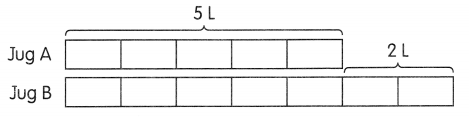Simon poured 7 liters of lemonade into Jug B.

Is Pete’s answer correct? Why or why not? If his answer is not correct, then find the correct answer. Draw a bar model to explain your reasoning.
Because jug A is having 5 litres and jug B is having 2 litres more than jug A.
Already Jug B has 3 litres after that Simon poured extra lemonade then it gets 7 litres. To know the correct amount of litres he poured we should subtract 7-3=4. He poured 4 litres extra to jug B, not 7 litres. So the answer is incorrect
It means 3+4=7 litres.Explanation: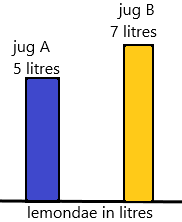Therefore, Simon poured 4 litres extra to jug B.

Look at the pictures.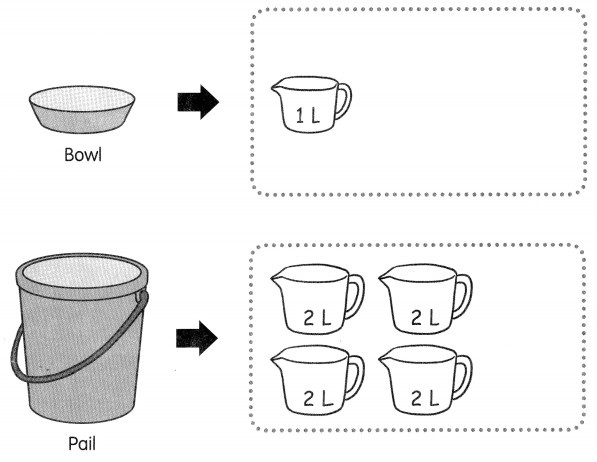a. How many bowls of water does it take to fill each 2-litre pitcher? ____ bowls
Explanation:
In the above picture, the bowl capacity is 1 litre. To fill each 2-litre pitcher 1 bowl for one time and 2nd bowl for the 2nd time. Therefore, 1 bowl +1 bowl=2 bowls are needed.

b. How many bowls of water does it take to fill the pail? ___ bowls
Explanation:
In the question asked, the number of bowls is needed to fill the pail.
To calculate this for each 1 bowl – 1 litre.
In the above 2nd picture, the pail can hold 8 litres of water. Count the 2-litre pitches. There are 4 pitches that can hold 2 litres each. Therefore, 4*2=8 litres.
we can fill pail up to X litres.
By applying cross-multiplication: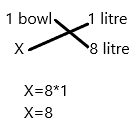So we use 8 bowls to fill the pail.

Problem Solving

Jasmine is a scientist. She found a new liquid called Liquid X. On the first day, she made 2 litres of Liquid X.
On the second day, she made 1 litre more of Liquid X than on the first day. Every day, she made 1 litre more than the day before.
What was the volume of Liquid X she made on Day 5?
Explanation:
The number of litres she made on the first day=2
The number of litres she made on the second day=2+1=3 (because she made 1 litre more than 1st day).
Likewise, she was making liquid X  1 litre more than the day before.
The number of litres she made on the third day=3+1=4 (day-by-day it increases by 1).
The number of litres she made on the fourth day=4+1=5
we need to calculate the fifth day:
The number of litres she made on the fifth day=5+1=6
Therefore, 6 litres of liquid X she made on fifth day.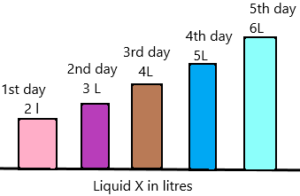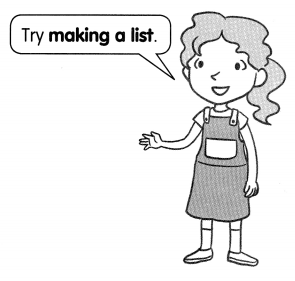Chapter Review/Test

Vocabulary

Fill in the blanks using these words.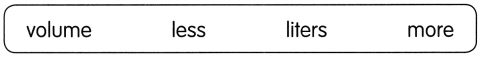Question 1.
The _________ is the amount of liquid in a container.
Definition of volume: A volume is simply defined as the amount of space occupied by any three-dimensional solid. These solids can be a cube, a cuboid, a cone, a cylinder or a sphere.
Unit of volume:
The volume of a solid is measured in cubic units. For example, if dimensions are given in meters, then the volume will be in cubic meters. This is the standard unit of volume in the International System of Units (SI). Similarly, other units of volume are cubic centimetres, cubic feet, cubic inches, etc.
Volume formula:
The formula to calculate the volume of a solid in a three-dimensional space is to find the product of dimensions. Basically, the volume is equal to the product of the area and height of the shape.
Volume =  Base Area x Height
For shapes having flat surfaces such as cube and cuboid, it is easy to find the volume. But for curved shapes like cone, cylinder and sphere, we have to consider the dimensions of its curved surface as well, such as radius or diameter.

Question 2.
The volume of a liquid can be measured in ____
Explanation:
The volume of liquid:
Basically, the volume of a liquid is measured in litres, where 1 litre is equal to 1000 cubic centimetres.
1 liter = 1000 cubic centimeters = 0.001 cubic meters
Hence,
1 cubic meters = 1000 liters
Also, to measure the volume of a small amount of liquid, we use millilitres.
1 milliliters = 0.001 liter = 1 cubic centimeters
The volume of liquid is also commonly measured in gallons.
1 liter = 0.264172 US liquid gallon.

Question 3.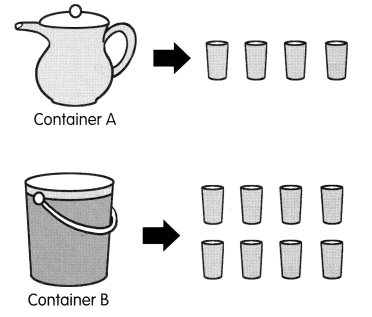a. Container A has _________ water than Container B.
Explanation:
Observe the above picture, container A can hold 4 glasses of water.

b. Container B has _________ water than Container A.
Explanation:
Observe the above picture, container B can hold 8 glasses of water which is more than container A. That’s why container B is more water than container A.

Concepts and Skills

Fill in the blanks.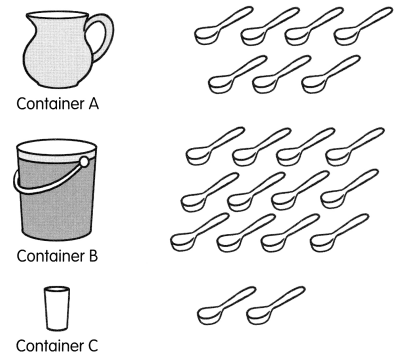Question 4.
Container _________ contains the least amount of water.
Explanation: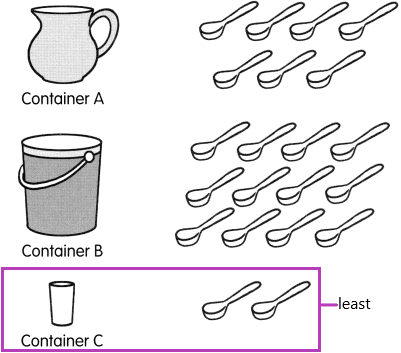Observe the picture, the container c has the least amount of water.
The volume of liquid:
– Basically, the volume of a liquid is measured in litres, where 1 litre is equal to 1000 cubic centimetres.
– 1 liter = 1000 cubic centimeters = 0.001 cubic meters
Hence,
– 1 cubic meters = 1000 liters
– Also, to measure the volume of a small amount of liquid, we use millilitres.
– 1 milliliters = 0.001 liter = 1 cubic centimeters
– The volume of liquid is also commonly measured in gallons.
– 1 liter = 0.264172 US liquid gallon.

Question 5.
Container B contains _________ more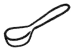water than the Container A.
Explanation:
The spoons of water in container B=12
The spoons of water in container A=7
The number of spoons container B is more than container A=X
Subtract the container B and container A
X=12-7
X=5

Find the volume of water.

Question 6.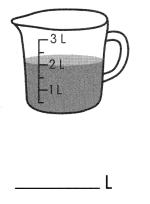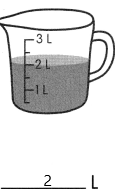volume of liquid:
– Basically, the volume of a liquid is measured in litres, where 1 litre is equal to 1000 cubic centimetres.
– 1 liter = 1000 cubic centimeters = 0.001 cubic meters
Hence,
– 1 cubic meters = 1000 liters
– Also, to measure the volume of a small amount of liquid, we use millilitres.
– 1 milliliters = 0.001 liter = 1 cubic centimeters
– The volume of liquid is also commonly measured in gallons.
– 1 liter = 0.264172 US liquid gallon.
To calculate the volume of liquid:
We need to know some key points which I had written in the below problem (7). Read once carefully and understand.
Formula:
The volume of sample=final water level-initial water level
volume of water=2-0
The volume of ware=2 litres.

Question 7.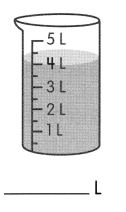Explanation:
1. Demonstrate what students will do by pouring water from a cup into a 100-mL graduated cylinder until it reaches a height that will cover the sample. This is the “initial water level.”
2. Tell students that the surface of the water in a tube may not be completely flat. Instead, the surface may curve in a shallow U-shape called the meniscus. When measuring, read the line just at the bottom of the meniscus.
3. Tilt the graduated cylinder and slowly slide the sample into the water. Hold the graduated cylinder upright. Record the level of the water. Point out that this is the “final water level.”
4. Tell students that you want to find out how much the water level changed. Subtract the initial water level from the final water level to find the volume of the rod.
5. Volume of sample = final water level − initial water level.
the volume of wate=4-0
the volume of water=4.

Find the volume of water.

Question 8.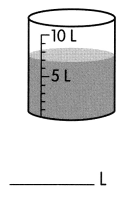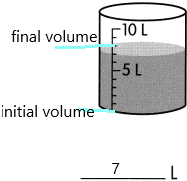The volume of water formula:
The volume of sample= final volume of water – an initial volume of water
Volume of water=7-0
volume of water=7.

Question 9.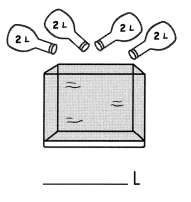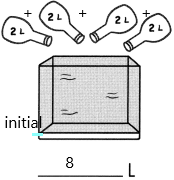Explanation:
It is like the volume of the cube.
Now a cube can hold 8 litres of water.
Here the initial point is 0 and the final point is 8.
How the final point is 8? will explain below.
2+2+2+2=8.
By applying the volume of water formula:
the volume of sample=final point of water-initial point of water.
volume of water=8-0
the volume of water=8.

Problem Solving

Solve.

Question 10.
Mr. Gomez makes 7 liters of apple juice for a party. He also makes 4 liters of orange juice. How much juice does he make for the party?
He makes ____ litres of juice for the party.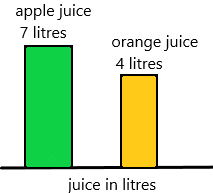Explanation:
The number of litres Gomez makes apple juice=7
The number of litres he makes orange juice=4
The total number of litres he makes juice for the party=X
X=7+4
X=11
Therefore, he makes 11 litres of juice for the party.

Question 11.
A tank has 13 litres of water. Lila uses 5 litres of water from the tank. How much water is left in the tank?
_____ litres of water is left in the tank.
Explanation: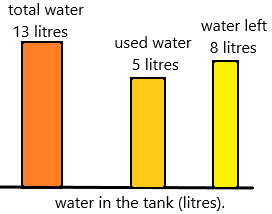The total number of litres a tank can hold=13
The number of litres Lila uses water from the tank=5
The number of litres of water left in the tank=X
Subtract total number of litres-used water
X=13-5
X=8
Therefore, 8 litres of water left in the tank.

Question 12.
12 litres of water are in a barrel. Mr Lopez pours 3 more liters of water into the barrel. Then he uses 7 liters of water from the barrel to water trees. How much water is left in the barrel?
____ litres of water is left in the barrel.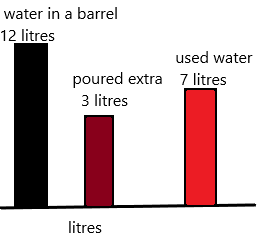Explanation:
The number of litres of water is present in barrel=12
The number of litres he pours extra into the barrel=3
The total number of litres=13+3=16 litres.
The number of litres he used=7
The number of litres of water left in the tank=X
X=16-7
X=9.
Therefore, 9 litres are left in the barrel.

Question 13.
A tub can hold 15 litres of water. 2 pails with 6 litres of water in each pail are poured into the tub. How much more water is needed to fill the tub?
___ more litres of water are needed to fill the tub.